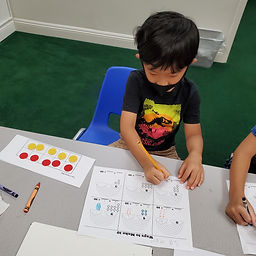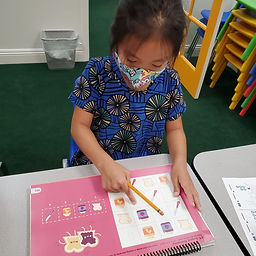## Ms. Raejean

### Target 1​

###### Lesson Type:

New

Number Operation

:

Computation

Identify all the two addend equations that produce the numbers one to ten.

###### 1:

Decompose the number zero to ten into all the combinations of quantities that produce the targeted number.

###### 2:

Understand that numbers can be derived in multiple ways.

2nd

###### Vocabulary:

Ten Frames, Counters

Activities:

• Students broke apart the numbers 1-10 to find all of the combinations to make them. This builds their number sense. We discussed how there is more than 1 way to find each of the numbers. (Ex. 1 = 0 + 1 or 1 + 0)
• Students used "Ten Frames" and Counters to find different ways to make 10. (0 + 10, 1 + 9, 2 + 8, 3 + 7, 4 + 6, 5 + 5) Students recorded the different combinations to make 10 on the "Ways to Make 10" sheet. Students proceeded to find the different ways to make the numbers 1 - 9.### Home Exploration

###### Guiding Questions:## Absent Students:

### Target 2

:

###### 1:

Use the given information in a problem to identify the correct answer.

###### 2:

Use the given information to determine which is not the correct answer.

2nd

###### Vocabulary:

Logic Reasoning

Activities:

• Students used their understanding of reading a grid (rows & columns) to determine which image was missing from the grid.
• Students also used the given clues/information to determine which pet belongs to each friend.### Home Exploration

###### Guiding Questions:### Target 3

:

###### Vocabulary:

Activities:### Home Exploration